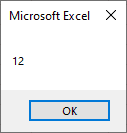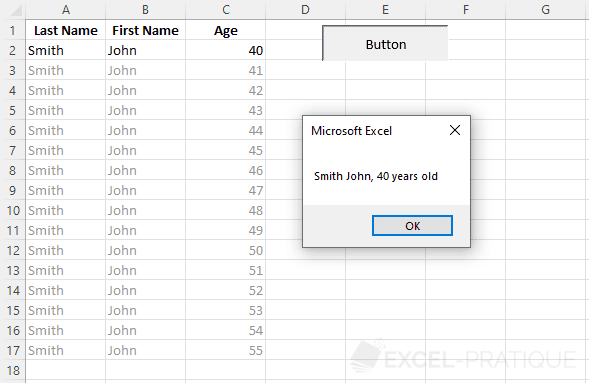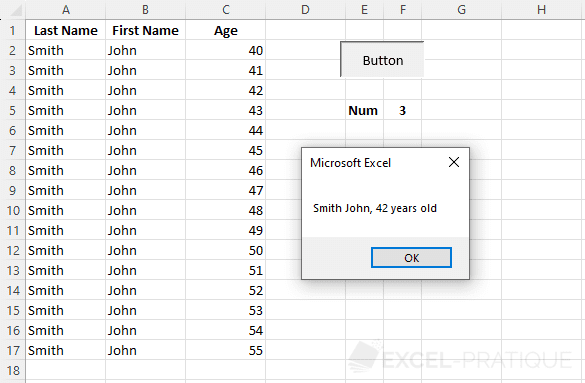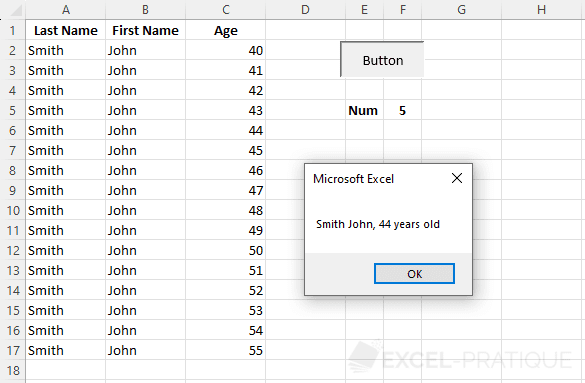# VBA course: variables

Variables allow to store all sorts of data.

Here is a first example:

``````'Display of the value of the variable in a dialog box
Sub variables()

'Declaration of the variable
Dim myVariable As Integer

'Attribution of a value to the variable
myVariable = 12

'Display of the value of myVariable in a MsgBox
MsgBox myVariable

End Sub``````

This first line of code is the declaration of the variable (generally placed at the beginning of the procedure).

``Dim myVariable As Integer``
• Dim: variable declaration
• myVariable: name chosen for this variable (no spaces)
• As: declaration of the variable type
• Integer: variable type

Declaring your variables is not mandatory but recommended. It allows to find your way around more easily, can help in some cases to solve problems more easily, etc. It's better to get into the habit of declaring your variables correctly.

The type of the variable indicates the nature of its content (text, numbers, date, etc.).

A value is then given to this variable:

``myVariable = 12``

And finally, the value of the variable is displayed in a dialog box:

``MsgBox myVariable``

MsgBox displays a value in a dialog box (the dialog boxes will be detailed in a few lessons).

The result of this code:If for now you do not understand the benefit of using variables, rest assured, the examples discussed in the upcoming lessons will demonstrate their usefulness.

## Variable types

NameTypeDetailsSymbol
ByteNumericInteger number from 0 to 255.
IntegerNumericInteger number from -32'768 to 32'767.%
LongNumericInteger number from - 2'147'483'648 to 2'147'483'647.&
CurrencyNumericFixed decimal number from -922'337'203'685'477.5808 to 922'337'203'685'477.5807.@
SingleNumericFloating point number from -3.402823E38 to 3.402823E38.!
DoubleNumericFloating point number from -1.79769313486232E308 to 1.79769313486232E308.#
StringTextText.\$
DateDateDate and time.
BooleanBooleanTrue or False.
ObjectObjectObject.
VariantAllAny data type (default type if the variable is not declared).

Some examples with different types:

``````'Example: integer number
Dim varInteger As Integer
varInteger = 12345

'Example: decimal number
Dim varDecimal As Single
varDecimal = 123.45

'Example: text
Dim varText As String
varText = "Excel-Pratique.com"

'Example: date
Dim varDate As Date
varDate = "06/12/2023"

'Example: true/false
Dim varBoolean As Boolean
varBoolean = True

'Example: object (Worksheet object for this example)
Dim varSheet As Worksheet
Set varSheet = Sheets("Sheet2") 'Set => assignment of a value to an object variable

'Example of using the object variable: activating the sheet
varSheet.Activate``````

The symbols indicated in the table above are used to shorten variable declarations.

For readability, they will not be used in the lessons but here is an example:

``````Dim example As Integer
Dim example%``````

These two lines are identical.

It is possible to enforce variable declarations by placing Option Explicit at the very beginning of the module (an error will be generated if a declaration is forgotten).

## Practical Example

We will now create step by step a macro that will retrieve the name in cell A2, the first name in cell B2, the age in cell C2 and will display them in a dialog box.

Source file: variables-exercise.xlsm

Let's start by declaring the variables (on the same line, separated by commas):

``````Sub variables()

'Declaration of variables
Dim name As String, firstname As String, age As Integer

End Sub``````

Then assign the cell values to the variables:

``````Sub variables()

'Declaration of variables
Dim name As String, firstname As String, age As Integer

'Values of variables
name = Cells(2, 1)
firstname = Cells(2, 2)
age = Cells(2, 3)

End Sub``````

And finally, display the result in the dialog box by concatenating the values with & (like in Excel formulas):

``````Sub variables()

'Declaration of variables
Dim name As String, firstname As String, age As Integer

'Values of variables
name = Cells(2, 1)
firstname = Cells(2, 2)
age = Cells(2, 3)

'Dialog box
MsgBox name & " " & firstname & ", " & age & " years old"

End Sub``````

Which gives us:We will now try to display in the dialog box the line of the table corresponding to the number indicated in cell F5.

Here is the goal:Take a moment to make this change yourself before moving on to the solution a little further down...

.
.
.
.
.
.
.
.
.
.
.
.
.
.
.
.
.
.
.
.
.
.
.
.
.
.
.
.
.
.

The solution:

``````Sub variables()

'Declaration of variables
Dim name As String, firstname As String, age As Integer, lineNumber As Integer

'Values of variables
lineNumber = Range("F5") + 1
name = Cells(lineNumber, 1)
firstname = Cells(lineNumber, 2)
age = Cells(lineNumber, 3)

'Dialog box
MsgBox name & " " & firstname & ", " & age & " years old"

End Sub``````

A variable lineNumber has been added:

``````'Declaration of variables
Dim name As String, firstname As String, age As Integer, lineNumber As Integer``````

The variable then takes the value of cell F5 to which we add 1 (not to consider the first line which contains the table titles).

The variable lineNumber will therefore have the value of the line number of the cells we are interested in:

``lineNumber = Range("F5") + 1``

All that remains is to replace the line numbers in Cells with our variable:

``````name = Cells(lineNumber, 1)
firstname = Cells(lineNumber, 2)
age = Cells(lineNumber, 3)``````

Our macro now displays the line of the table we are interested in.Note that we can reduce this entire procedure to one line:

``````Sub variables()
MsgBox Cells(Range("F5")+1,1) & " " & Cells(Range("F5")+1,2) & ", " & Cells(Range("F5")+1,3) & " years old"
End Sub``````

The code works correctly, but it is much less readable than the previous one and more difficult to maintain in the future (the codes will therefore not be reduced in the lessons in order to facilitate understanding).